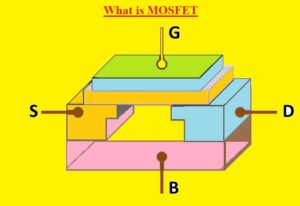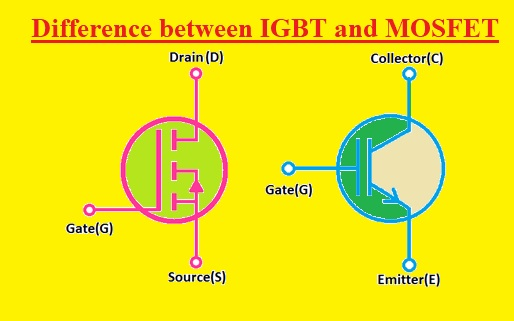Hello, readers welcome to the new post. We will learn Difference between IGBT and MOSFET.  These two components are transistors that are used in electronic circuits. These two modules are regulated through the use of volts.

In this post, we will cover their different parameters to find the differences. So let’s get started Difference between IGBT and MOSFET

### Difference between IGBT and MOSFET

#### What is IGBT

• IGBTs are also three terminal electronic components. Its pinout is emitter-collector and gate. It has a gate then base like BJT.
It is used for high-performance control operations as well as high-speed switching. It was the first time he had been sued in the 1980s
• This transistor comes with dual functions of MOSFET and BJT. It is gate-driven like a driven MOSFET and has the current-voltage characteristics of a BJT.
• It can therefore be used for high current operations as well as for high power circuits
• Its internal circuit is a combination of 2 transistors and a MOSFET and its output is a combination of PNP NPN and MOSFET transistors.
• This transistor limits the smaller value of the transistor saturation volts to the high value of the input impedance at the MOSET switching speed.### IGBT Types

• There are 2 main types of IGBTs based on the N+buffer layer, the IGBT category has n+buffer layers known as Punch through IGBTs, and others are called non-punch through IGBTs.
• Based on the different characteristics, these two IGBTs are called symmetrical and unsymmetrical IGBTs.
• For symmetrical IGBTs, there are equal forward and reverse breakdown volts.
• While asymmetric IGBTs have reverse breakdown volts less than forward breakdown volts
• Symmetric IGBT is used in AC circuits and Asymmetric IGBT is used for DC circuits

### What is MOSFET

• A MOSFET is also known as a metal oxide silicon transistor or MOS is a category of IGFET or insulated gate FET that is made by thermally oxidizing a semiconductor material usually silicon.
• The isolated gate voltage calculates the electrical conductivity of the transistor this behavior of conductance changes with quantity if the given voltage can be used in amplifiers and switching circuits.
In 1959, MOSFET was first created by Egyptian engineer Mohamed M.Atalla and Dawon Kahng who was Korian engineer.
• This electronic component is most commonly used in electronic circuits, and nearly  (1.3 × 1022) MOSFETs have been created from 1960 to 2018.
• It is also very often used in digital, analog circuits and power devices.
• The main advantages of MOSFETs are that no input current is needed to regulate the load current as required in a BJT.
• The voltage applied to the gate of the E-MOSFET can increase the conductance from the off state.
While for a D-MOSFET the gate voltage can decrease the conductance from the on state.
The switching speed of this module is high, its size is small, it consumes less power.### IGBT vs MOSFET• The full form of IGBT is an insulated gate bipolar transistor and MOSFET’s full form is a Metal oxide semiconductor field effect transistor
• IGBT has three terminals and MOSFET has 4 terminals
• Names of IGBT pins are emitter gate transistor and MOSFET are the source, gate-drain, and substrate
• IGBT comes with a PN junction and MOSFET not have a PN junction
• IGBT is used for mid to high level of current and MOSFET handle low to medium current
• MOSFET handles less power than IGBT
• MOSFET used for high frequency than IGBT
• FOrwards volts for IGBT are less than the MOSFET
• Off time for MOSFET is less than IGBT
• The switching speed of IGBT is less than MOSFET
• For handling of transients current and volts, IGBT is used than MOSFET
• Saturation volts of MOSFET is less than IGBT
• Prices of IGBT is higher than the MOSFET

That is all about the Difference between IGBT and MOSFET all details has explained. If you have any queries ask in the comments. Thanks for reading have a nice day